library(magrittr)
library(grid)
library(ComplexHeatmap)
library(Seurat)
library(ggplot2)
library(ggsector)

## Use original coordinates with grid.polygon

### coordinates of single sector

type of percent, start = 0, r_start = 0

tmp_df <- sector_df(x = 0.5, y = 0.5, theta = 25, r = 0.4, start = 0, r_start = 0)
#>           x         y
#> 1 0.5000000 0.5000000
#> 2 0.5000000 0.9000000
#> 3 0.5251162 0.8992107
#> 4 0.5501333 0.8968459
#> 5 0.5749525 0.8929149
#> 6 0.5994760 0.8874333
grid.newpage()
grid.polygon(
tmp_df$x, tmp_df$y,
vp = viewport(height = unit(1, "snpc"), width = unit(1, "snpc"))
)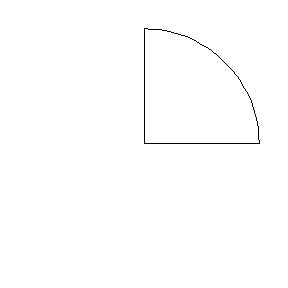type of percent, start = 50, r_start = 0.2

tmp_df <- sector_df(x = 0.5, y = 0.5, theta = 25, r = 0.4, start = 50, r_start = 0.2)
#>           x         y
#> 1 0.5000000 0.1000000
#> 2 0.4748838 0.1007893
#> 3 0.4498667 0.1031541
#> 4 0.4250475 0.1070851
#> 5 0.4005240 0.1125667
#> 6 0.3763932 0.1195774
grid.newpage()
grid.polygon(
tmp_df$x, tmp_df$y,
vp = viewport(height = unit(1, "snpc"), width = unit(1, "snpc"))
)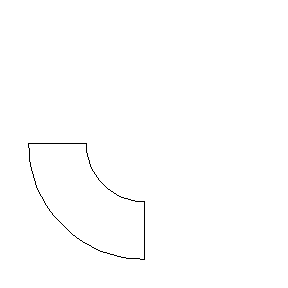type of degree, start = 90, r_start = 0

tmp_df <- sector_df(
x = 0.5, y = 0.5, theta = 180, r = 0.4,
start = 90, r_start = 0, type = "degree"
)
#>           x         y
#> 1 0.5000000 0.5000000
#> 2 0.9000000 0.5000000
#> 3 0.8999391 0.4930190
#> 4 0.8997563 0.4860402
#> 5 0.8994518 0.4790656
#> 6 0.8990256 0.4720974
grid.newpage()
grid.polygon(
tmp_df$x, tmp_df$y,
vp = viewport(height = unit(1, "snpc"), width = unit(1, "snpc"))
)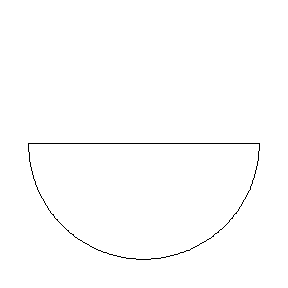type of degree, start = 180, r_start = 0.2

tmp_df <- sector_df(
x = 0.5, y = 0.5, theta = 180, r = 0.4,
start = 270, r_start = 0.2, type = "degree"
)
#>           x         y
#> 1 0.1000000 0.5000000
#> 2 0.1000609 0.5069810
#> 3 0.1002437 0.5139598
#> 4 0.1005482 0.5209344
#> 5 0.1009744 0.5279026
#> 6 0.1015221 0.5348623
grid.newpage()
grid.polygon(
tmp_df$x, tmp_df$y,
vp = viewport(height = unit(1, "snpc"), width = unit(1, "snpc"))
)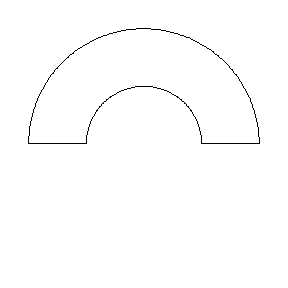### Coordinates of Multiple Sectors

tmp_df <- sector_df_multiple(
x = c(0.2, 0.5, 0.8),
theta = c(25, 50, 75),
r = 0.15,
start = c(75, 50, 100),
r_start = c(0, 0.05, 0.1),
type = "percent"
)
#>            x         y group
#> 1 0.20000000 0.5000000     1
#> 2 0.05000000 0.5000000     1
#> 3 0.05029599 0.5094186     1
#> 4 0.05118279 0.5188000     1
#> 5 0.05265691 0.5281072     1
#> 6 0.05471253 0.5373035     1
grid.newpage()
grid.polygon(
tmp_df$x, tmp_df$y,
id = tmp_df$group, vp = viewport(height = unit(1, "snpc"), width = unit(1, "snpc")), gp = gpar( fill = 3:1, col = 1:3 ) )## Use ggsector with grid ### sectorGrob sectorGrob with units of “cm” and type of “degree” grid.newpage() gp <- sectorGrob( x = unit(c(3, 5, 7), "cm"), y = unit(c(3, 5, 7), "cm"), theta = c(90, 180, 270), r = 1, start = c(180, 180, 270), r_start = c(0.6, 0.3, 0), type = "degree", group = factor(1:3, levels = c(2, 3, 1)), gp = gpar(fill = c("green", "red", "blue")) ) grid.draw(gp)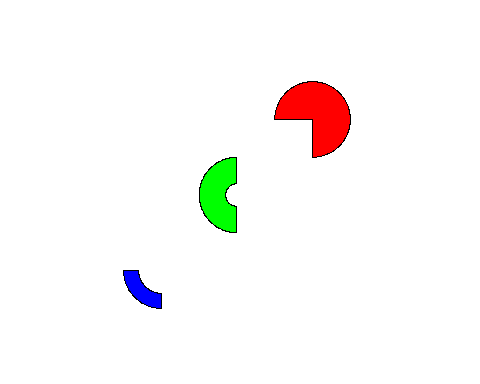### grid.sector grid.sector with units of “npc” and type of “percent” grid.newpage() grid.sector( x = c(0.1, 0.5, 0.9), y = c(0.9, 0.6, 0.1), theta = c(25, 50, 90), r = .1, start = c(25, 50, 100), r_start = c(0.06, 0.03, 0), type = "percent", group = factor(1:3, levels = c(2, 3, 1)), gp = gpar(col = c("green", "red", "blue"), fill = 2:4), default.units = "npc" )## Use ggsector with ComplexHeatmap ### Prepare data library(magrittr) library(ComplexHeatmap) t0 <- cor(mtcars) %>% set_colnames(paste("y_", colnames(.))) %>% set_rownames(paste("x_", rownames(.))) mat <- abs(t0) mat[1:5, 1:5] #> y_ mpg y_ cyl y_ disp y_ hp y_ drat #> x_ mpg 1.0000000 0.8521620 0.8475514 0.7761684 0.6811719 #> x_ cyl 0.8521620 1.0000000 0.9020329 0.8324475 0.6999381 #> x_ disp 0.8475514 0.9020329 1.0000000 0.7909486 0.7102139 #> x_ hp 0.7761684 0.8324475 0.7909486 1.0000000 0.4487591 #> x_ drat 0.6811719 0.6999381 0.7102139 0.4487591 1.0000000 ### cell_fun + viewport Realized by modifying the [grid::viewport()] with cell_fun, the sector can be set with a fixed width and height set.seed(1) Heatmap( mat, name = "vp", rect_gp = gpar(type = "none"), cell_fun = function(j, i, x, y, width, height, fill) { grid.rect( x = x, y = y, width = width, height = height, gp = gpar(col = "grey", fill = NA) ) grid.sector( theta = mat[i, j] * 100, r = 0.5, start = mat[i, j] * 100 * runif(1), r_start = mat[i, j] * 0.49 * runif(1), vp = viewport(x, y, width, height), gp = gpar(fill = fill, col = "transparent") ) }, width = unit(.7, "snpc"), height = unit(.7, "snpc") )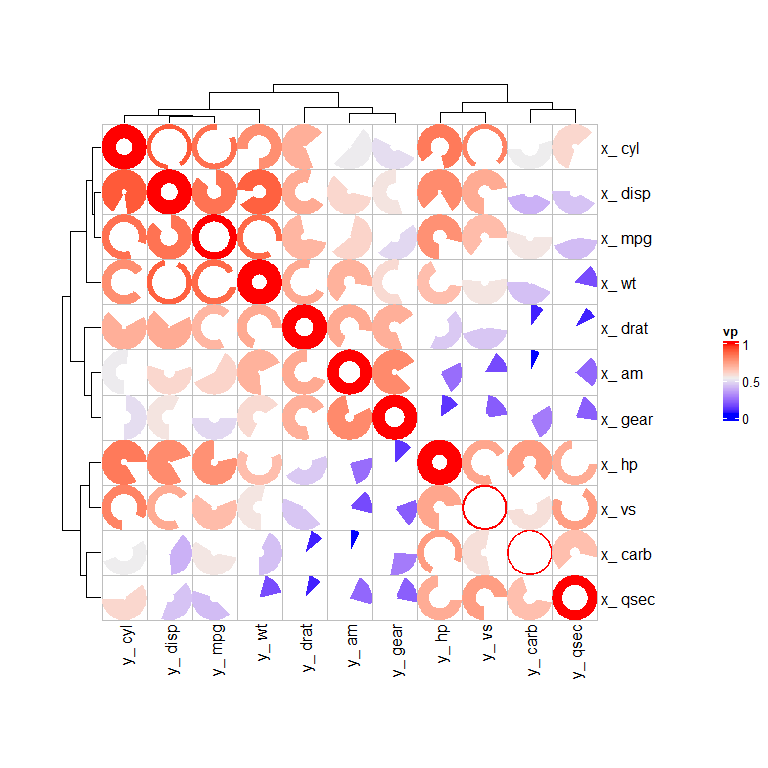### cell_fun + xy Realized in the form of coordinates + radius with cell_fun. # The default viewport locks the horizontal and vertical axes # so that the sector does not deform, which needs to be removed here. # The radius 'r' is half the min(length, width). set.seed(2) Heatmap( mat, name = "xy + r", rect_gp = gpar(type = "none"), cell_fun = function(j, i, x, y, width, height, fill) { grid.rect( x = x, y = y, width = width, height = height, gp = gpar(col = "grey", fill = NA) ) r <- as.numeric(min(width, height)) / 2 grid.sector( x, y, theta = mat[i, j] * 100, r = r, start = mat[i, j] * 100 * runif(1), r_start = mat[i, j] * r * 0.9 * runif(1), vp = NULL, gp = gpar(fill = fill, col = "transparent") ) }, width = unit(.7, "snpc"), height = unit(.7, "snpc") )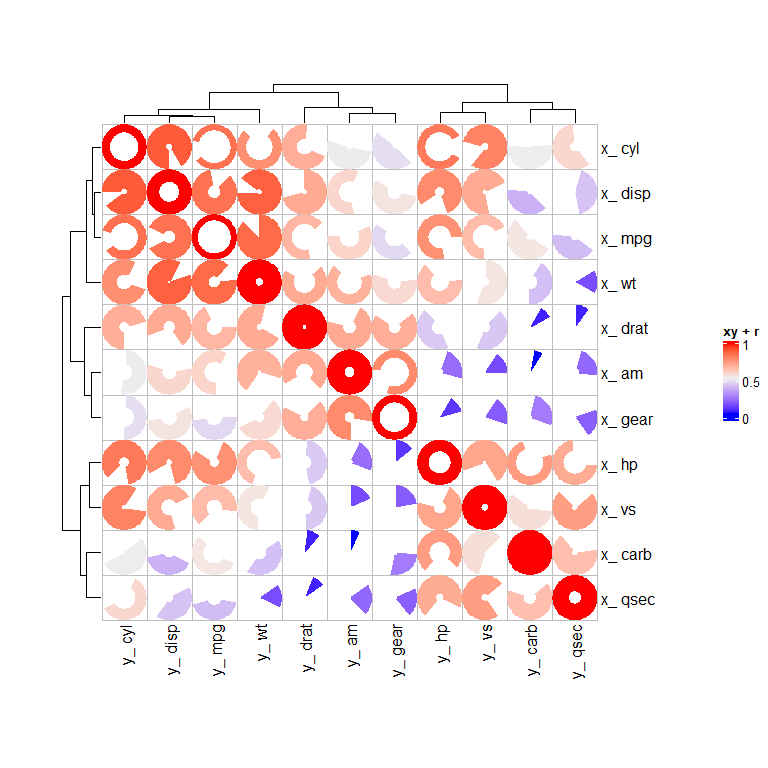### layer_fun + xy Realized With layer fun # The input matrix needs to be extracted with pindex(mat, i, j) set.seed(3) Heatmap( mat, name = "layer", rect_gp = gpar(type = "none"), layer_fun = function(j, i, x, y, width, height, fill) { grid.rect( x = x, y = y, width = width, height = height, gp = gpar(col = "grey", fill = NA) ) r <- as.numeric(min(width, height)) / 2 grid.sector( x, y, theta = pindex(mat, i, j) * 100, r = r, start = pindex(mat, i, j) * 100 * runif(nrow(mat) * ncol(mat)), r_start = pindex(mat, i, j) * r * 0.9 * runif(nrow(mat) * ncol(mat)), vp = NULL, gp = gpar(fill = fill, col = "transparent") ) }, width = unit(.7, "snpc"), height = unit(.7, "snpc") )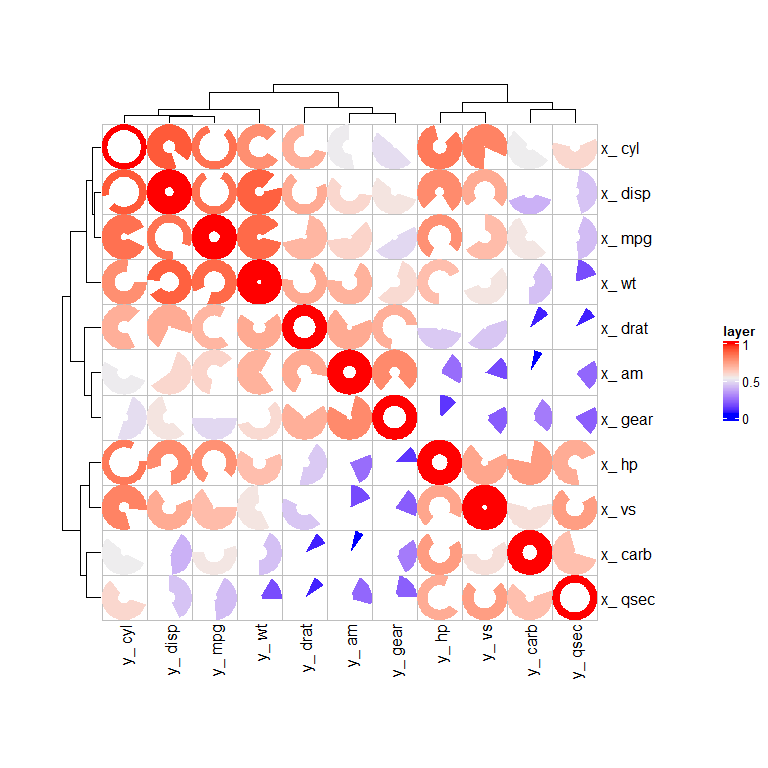## Use ggsector with ggplot2 ### prepare data library(ggsector) library(reshape2) df <- cor(mtcars)[1:3, 1:5] %>% abs() %>% melt(varnames = c("x", "y")) ## Note, for better display effect, please always add coord_fixed() ## Note, for better display effect, please always add coord_fixed() ## Note, for better display effect, please always add coord_fixed() ### theta The sector angle parameter, used in combination with the type parameter, the type parameter defaults to “percent”. When type = “percent”, the complete circle is a polygon composed of 100 scattered points, and theta takes a value of 0-100. When type = “degree”, the complete circle is a polygon composed of 360 scattered points, and theta takes a value of 0-360. ggplot(df) + ## type = "percent", theta = 0-100 geom_sector( aes(y, x, theta = value * 100), type = "percent", color = "blue", individual = TRUE ) + ## type = "degree", theta = 0-360 geom_sector( aes(y, x, theta = value * 360), type = "degree", color = "red", alpha = 0.5, individual = TRUE ) + coord_fixed() + theme_bw() + theme(axis.title = element_blank()) #> For better display effect, please add coord_fixed() #> For better display effect, please add coord_fixed()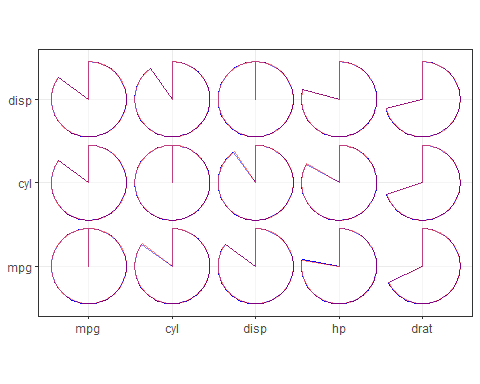Careful observation reveals: The sectors shapes in the two modes are not completely overlapped, this is because: when type = “percent”, the circumference is 100 scattered points, and the input value will be round() to an integer of 0-100, when type = “degree”, the circumference is 360 scattered points, and the input value will be round() to an integer of 0-360. The more circle points, the higher the precision, but also means the slower drawing speed. ### r Radius of the outer circle of the sector(0-0.5) ggplot(df) + geom_sector( aes(y, x, theta = value * 100), r = rep(c(0.15, 0.3, 0.45), 5), fill = 2, individual = TRUE ) + coord_fixed() + theme_bw() + theme(axis.title = element_blank()) #> For better display effect, please add coord_fixed()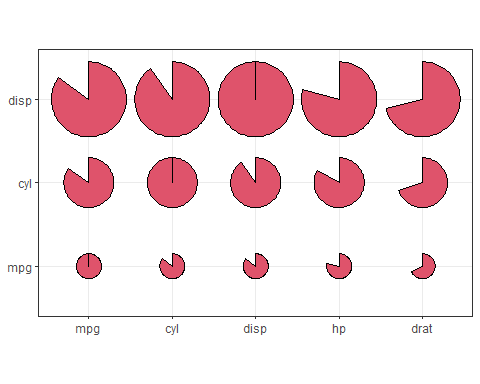### start Starting angle of sector. ggplot(df) + geom_sector( aes(y, x, theta = value * 100), start = rep(c(60, 40, 20), 5), fill = 2, individual = TRUE ) + coord_fixed() + theme_bw() + theme(axis.title = element_blank()) #> For better display effect, please add coord_fixed()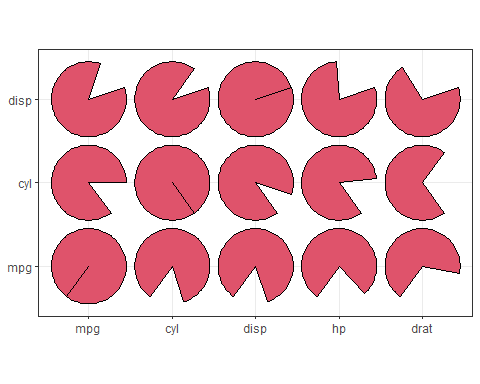### r_start The starting position parameter of the fan radius, the value is between 0 and the radius length, and the default is 0, which means drawing a fan shape. If it is greater than 0, it is to draw a sector, and the following are different displays ggplot(df) + geom_sector( aes(y, x, theta = value * 100), r_start = rep(c(0.15, 0.25, 0.35), 5), fill = 2, individual = TRUE ) + coord_fixed() + theme_bw() + theme(axis.title = element_blank()) #> For better display effect, please add coord_fixed()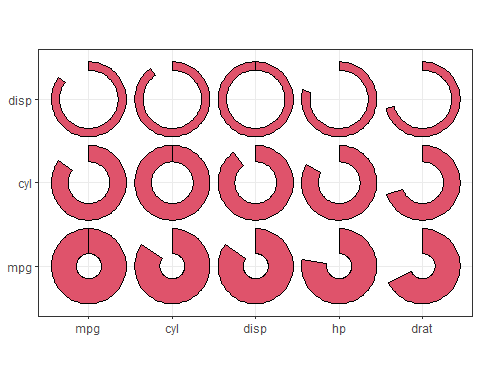### individual The default is FALSE, mainly to control whether to draw sector by sector with a single coordinate point or to draw as a whole on the drawing board in the form of vector. When individual = TRUE, draw one by one, the sector will not be deformed, but when there are too many sectors drawn, the overall drawing speed will be much slower. When individual = FALSE, drawing in vector form is faster, but it needs to be used with coord_fixed() or ratio to lock the aspect ratio of the drawing board, otherwise the sector will be deformed. #### individual with coord_fixed() For better display effect, please always add coord_fixed(). ##### individual = TRUE + coord_fixed() # x = x, y = y ggplot(rbind( cbind(df, t1 = 1), cbind(df[1:9, ], t1 = 2) )) + facet_wrap(~t1, ncol = 2) + geom_sector( aes(x, y), theta = 75, fill = 2, r = 0.5, individual = TRUE ) + coord_fixed() + theme_bw() + theme(axis.title = element_blank()) #> For better display effect, please add coord_fixed()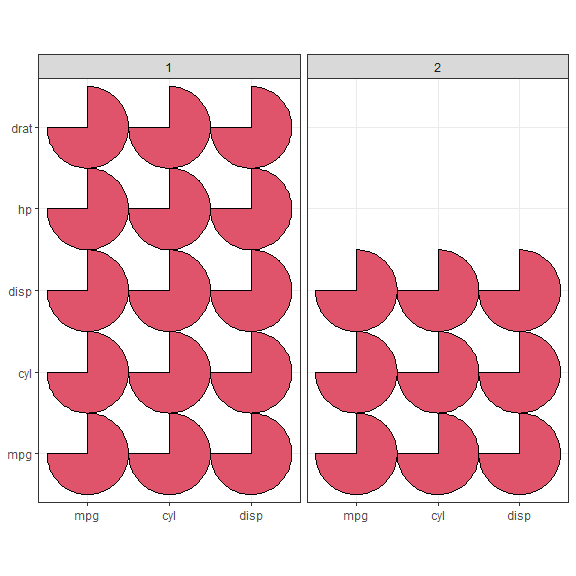# x = y, y =x ggplot(rbind( cbind(df, t1 = 1), cbind(df[1:9, ], t1 = 2) )) + facet_wrap(~t1, ncol = 2) + geom_sector( aes(y, x), theta = 75, fill = 2, r = 0.5, individual = TRUE ) + coord_fixed() + theme_bw() + theme(axis.title = element_blank()) #> For better display effect, please add coord_fixed()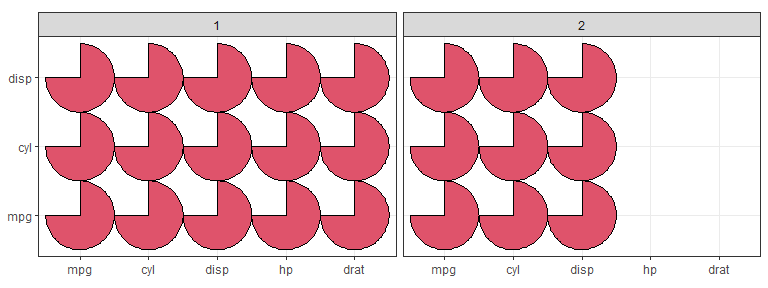##### individual = FALSE + coord_fixed() # x = x, y = y ggplot(rbind( cbind(df, t1 = 1), cbind(df[1:9, ], t1 = 2) )) + facet_wrap(~t1, ncol = 2) + geom_sector( aes(x, y), theta = 75, fill = 2, r = 0.5, individual = FALSE ) + coord_fixed() + theme_bw() + theme(axis.title = element_blank()) #> For better display effect, please add coord_fixed()# x = y, y =x ggplot(rbind( cbind(df, t1 = 1), cbind(df[1:9, ], t1 = 2) )) + facet_wrap(~t1, ncol = 2) + geom_sector( aes(y, x), theta = 75, fill = 2, r = 0.5, individual = TRUE ) + coord_fixed() + theme_bw() + theme(axis.title = element_blank()) #> For better display effect, please add coord_fixed()#### individual without coord_fixed() ##### individual = TRUE without coord_fixed() If you are in a special situation and cannot use coord_fixed(), then it is recommended that you use individual = TRUE and the r parameter to fine-tune. Also, to reduce the radius, you need to try it manually. # x = x, y = y ggplot(rbind( cbind(df, t1 = 1), cbind(df[1:9, ], t1 = 2) )) + facet_wrap(~t1, ncol = 2) + geom_sector( aes(x, y), theta = 75, fill = 2, r = 0.35, ## To reduce the radius, you need to try it manually individual = TRUE ) + theme_bw() + theme(axis.title = element_blank()) #> For better display effect, please add coord_fixed()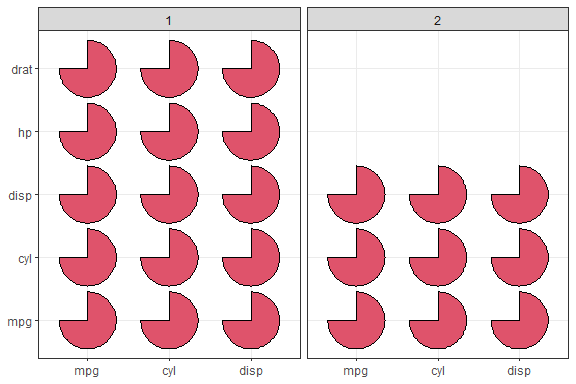# x = y, y =x ggplot(rbind( cbind(df, t1 = 1), cbind(df[1:9, ], t1 = 2) )) + facet_wrap(~t1, ncol = 2) + geom_sector( aes(y, x), theta = 75, fill = 2, r = 0.25, ## To reduce the radius, you need to try it manually individual = TRUE ) + theme_bw() + theme(axis.title = element_blank()) #> For better display effect, please add coord_fixed()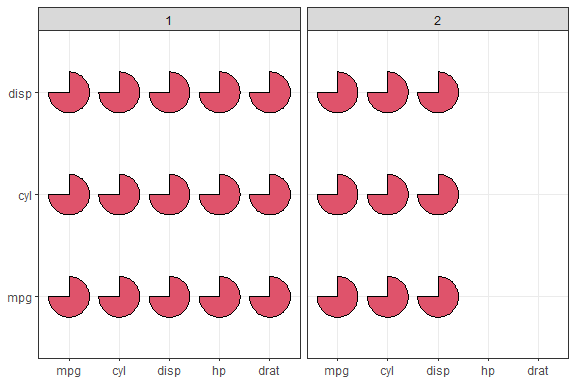##### individual = FALSE without coord_fixed() If you really want to use individual = FALSE without coord_fixed(), you might try the experimental parameter ratio' You need to manually adjust theratio value to prevent sector deformation. # x = x, y = y ggplot(rbind( cbind(df, t1 = 1), cbind(df[1:9, ], t1 = 2) )) + facet_wrap(~t1, ncol = 2) + geom_sector( aes(x, y), theta = 75, fill = 2, r = 0.5, ## You need to manually adjust the ratio value ## to prevent sector deformation. ratio = 1.6, individual = FALSE ) + theme_bw() + theme(axis.title = element_blank()) #> For better display effect, please add coord_fixed() #> #> This is not an error or warning, just a small reminder. #> The calculated optimal ratio value is: 1.625. #> The ratio value currently used is: 1.6. #> #> This is not an error or warning, just a small reminder. #> The calculated optimal ratio value is: 1.625. #> The ratio value currently used is: 1.6.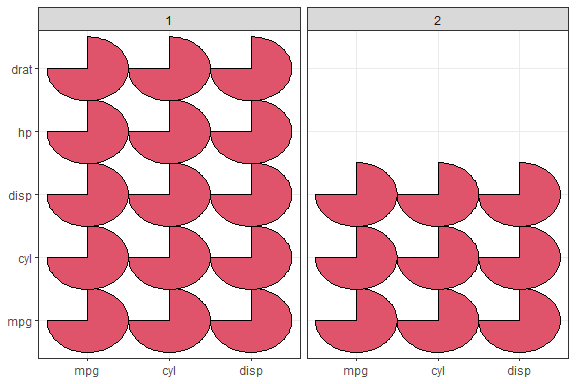# x = y, y =x ggplot(rbind( cbind(df, t1 = 1), cbind(df[1:9, ], t1 = 2) )) + facet_wrap(~t1, ncol = 2) + geom_sector( aes(y, x), theta = 75, fill = 2, r = 0.5, ## You need to manually adjust the ratio value ## to prevent sector deformation. ratio = 1.6, individual = FALSE ) + # coord_fixed() + theme_bw() + theme(axis.title = element_blank()) #> For better display effect, please add coord_fixed() #> #> This is not an error or warning, just a small reminder. #> The calculated optimal ratio value is: 0.615384615384616. #> The ratio value currently used is: 1.6. #> #> This is not an error or warning, just a small reminder. #> The calculated optimal ratio value is: 0.615384615384616. #> The ratio value currently used is: 1.6.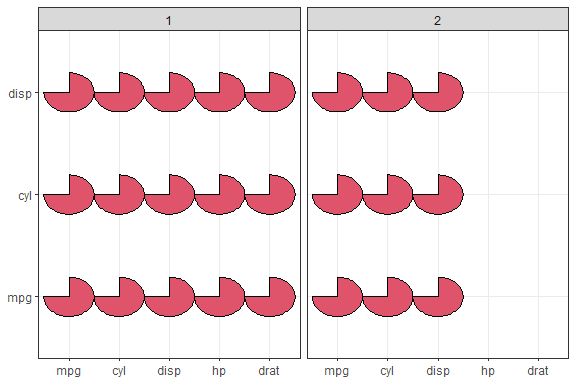## Use ggsector with Seurat Due to the large raw data of “pbmc”, only the code is shown here, but not run. Readers are invited to download the data and try it out. ## Download pbmc data from # https://cf.10xgenomics.com/samples/cell/pbmc3k/pbmc3k_filtered_gene_bc_matrices.tar.gz library(Seurat) path <- paste0(tempdir(), "/pbmc3k.tar.gz") file <- paste0(tempdir(), "/filtered_gene_bc_matrices/hg19") download.file( "https://cf.10xgenomics.com/samples/cell/pbmc3k/pbmc3k_filtered_gene_bc_matrices.tar.gz", path ) untar(path, exdir = tempdir()) pbmc.data <- Read10X(data.dir = file) pbmc <- CreateSeuratObject( counts = pbmc.data, project = "pbmc3k", min.cells = 3, min.features = 200 ) pbmc <- NormalizeData(pbmc) pbmc <- FindVariableFeatures(pbmc, selection.method = "vst", nfeatures = 2000) pbmc <- ScaleData(pbmc, features = rownames(pbmc)) pbmc <- RunPCA(pbmc) pbmc <- RunUMAP(pbmc, dim = 1:10) pbmc <- FindNeighbors(pbmc, dims = 1:10) pbmc <- FindClusters(pbmc, resolution = 1) pbmc <- FindClusters(pbmc, resolution = 0.5) mks <- tibble::tribble( ~type, ~marker, "Naive CD4+ T", "IL7R,CCR7", "CD14+ Mono", "CD14,LYZ", "Memory CD4+", "IL7R,S100A4", "B", "MS4A1", "CD8+ T", "CD8A", "FCGR3A+ Mono", "FCGR3A,MS4A7", "NK", "GNLY,NKG7", "DC", "FCER1A,CST3", "Platelet", "PPBP", ) %>% tidyr::separate_rows(marker, sep = ", *") %>% dplyr::distinct() # Dotplot DotPlot(pbmc, features = unique(markers$marker)) + coord_flip()

# contrast with DotPlot
SectorPlot(pbmc, markers$marker, features.level = unique(rev(markers$marker)))

SectorPlot(pbmc, markers$marker, group.by = "RNA_snn_res.1") # split plot # Assume a variable 'day', expressed as the number of days of cell development. set.seed(1) pbmc[["day"]] <- sample(1:3, ncol(pbmc), TRUE) SectorPlot(pbmc, markers$marker, group.by = "RNA_snn_res.0.5", split.by = "day")
SectorPlot(
pbmc, markers\$marker,
group.by = "day", split.by = "RNA_snn_res.0.5", nrow = 1
)`